Kerr Newman Metric

English Version
Yukterez
Beiträge: 207
Registriert: Mi 21. Okt 2015, 02:16

Kerr Newman MetricKerr-Newman, second order differential equations of motion for a charged particle and photons. Animations by Simon Tyran, Vienna (Yukterez)This is the english version.Deutschsprachige Version auf kerr.newman.yukterez.net und Yukipedia.←
Shadow of an extremal Kerr-Newman BH with a²+℧²=M², Angle of view: edge on. For other parameters see here. Raw material: Commons.←
Zoom with overlayed ergoshphere and horizon surfaces. Comparison with an uncharged Kerr black hole: click here←
Accretion disk around a spinning and charged BH with a=0.95, ℧=0.3, inner radius ri=isco, outer radius ra=10, viewpoint=89°←
Gravitylensed surface of a charged and rotating body with the same surface as the earth. a=0.95, ℧=0.3, r=1.01r+, viewpoint=89°←
Retrograde orbit of a charged particle (q=1) around a BH with spin & charge a=√¾ & ℧=⅓. v0 & i0: local initial velocity & inclination←
Prograde orbit of a neutral testparticle around a spinning and electrically charged black hole with spin a=0.9 and charge ℧=0.4←
Prograde orbit of a negatively charged testparticle around a spiining and positively charged black hole with the same parameters as above←
Nonequatorial and retrograde photon orbit around a spinning (a=½) and charged (℧=½) black hole, constant Boyer Lindquist radius←
Polar photon orbit around a spinning (a=0.5) and charged (℧=0.75) naked singularity, constant Boyer Lindquist radius←
Polar orbit (Lz=0) of a positively charged testpaticle (q=⅓) around a positively charged and spinning black hole (℧=a=0.7)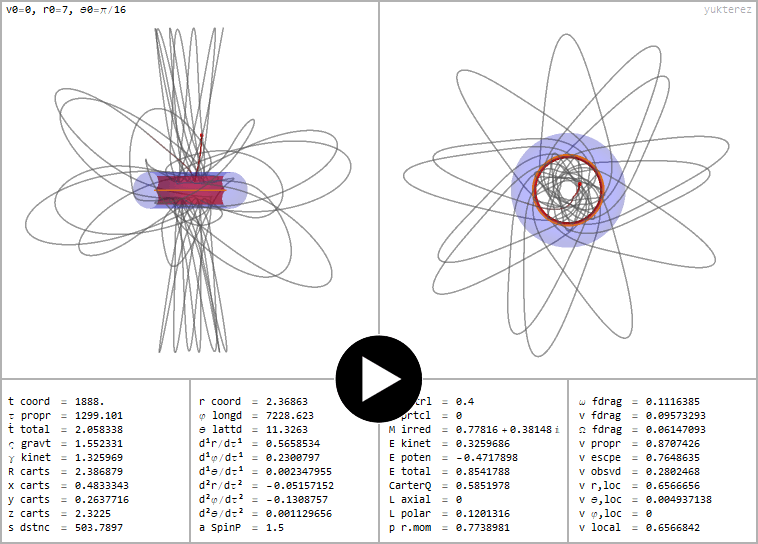←
Free fall of a neutral testparticle around a rotating and charged naked singularity with spin a=1.5 and electric charge ℧=0.4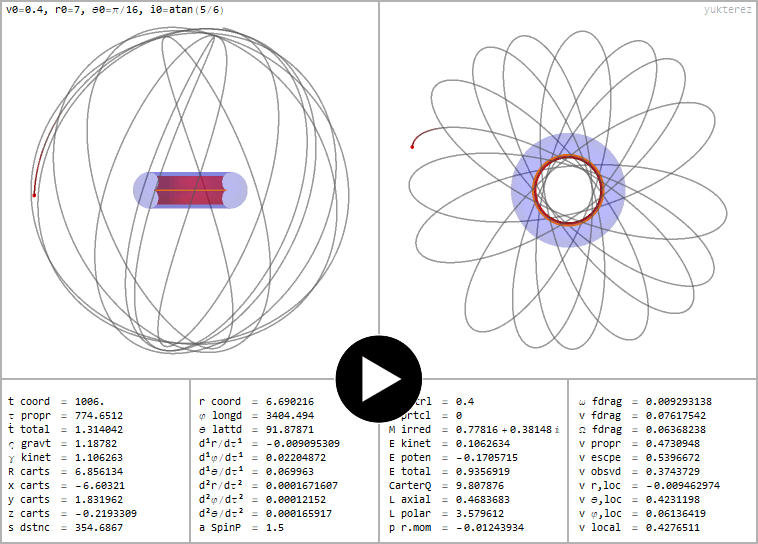←
Geodesic orbit around a naked Kerr Newman ringsingularity with the same spin and charge parameters as in the last example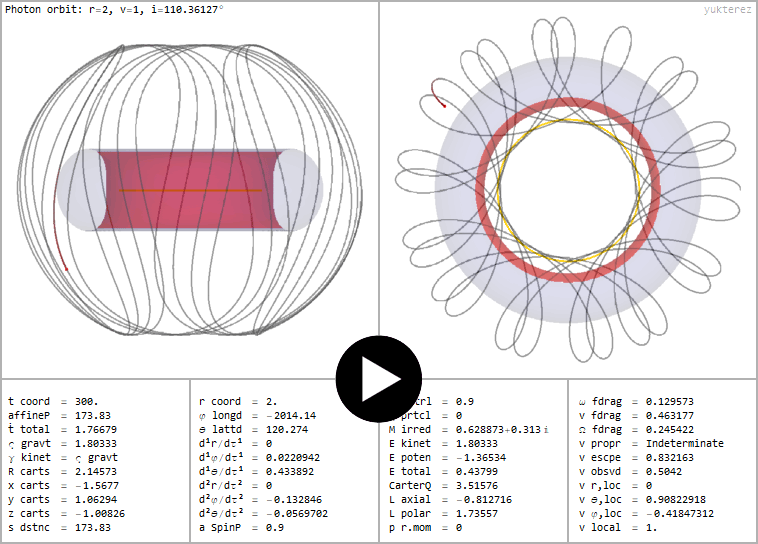←
Nonequatorial and retrograde photon orbit around a naked singularity spinning with a=0.9 and charged with ℧=0.9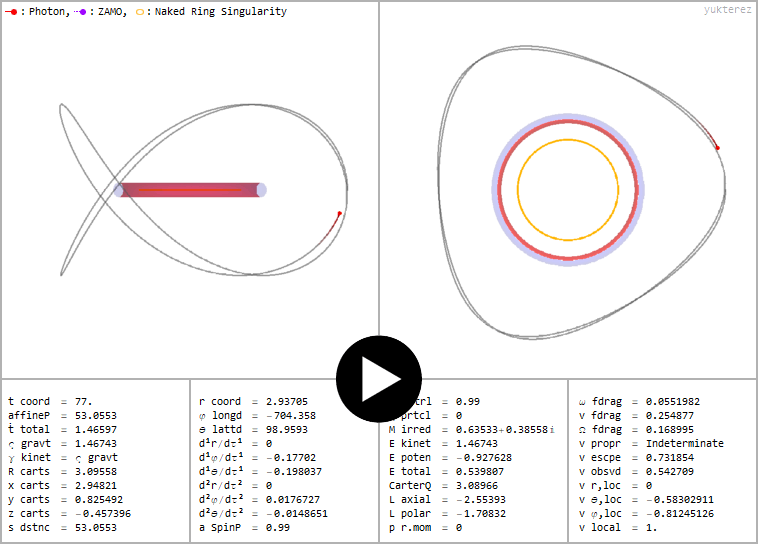←
Retrograde photon orbit around a naked singularity (a=0.99, ℧=0.99). Local equatorial inclination angle: -2.5rad=-143.23945°←
Stationary photon orbit (E=0) around a ringsingularity (a=½, ℧=1). Except at r=1, θ=90° v framedrag is <c everywhere, therefore no ergospheres.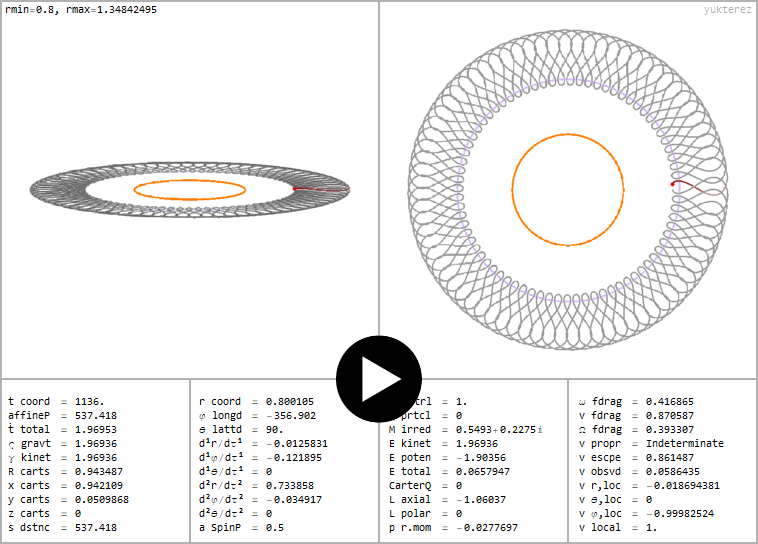←
Equatorial retrograde photon orbit, singularity at r=0→R=√(r²+a²)=a=½. Ergoring (green) at r=1, turning points at r=0.8 and r=1.3484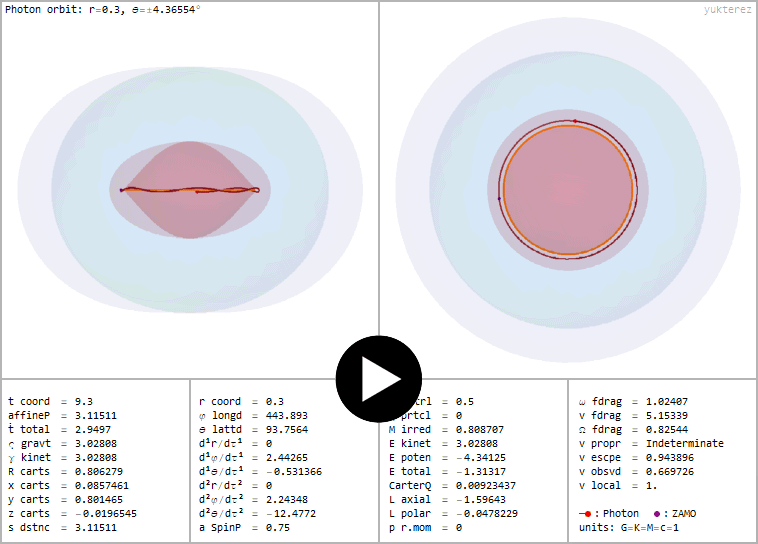←
Retrograde photon orbit of the third kind insinde a spinning (a=0.75) and charged (℧=0.5) black hole, constant BL radius←
Orbit of a negatively charged particle inside a positively charged Reissner Nordström black hole (also see Dokuchaev, Fig. 1)Simon Tyran aka Симон Тыран @ minds || vk || 1984 || wikipedia || stackexchange || wolframYukterez
Beiträge: 207
Registriert: Mi 21. Okt 2015, 02:16

Kerr Newman MetricLine element in Boyer Lindquist coordinates, metric signature (+,-,-,-):Shorthand terms:with the spin parameter â=Jc/G/M or in dimensionless units a=â/M, the specific electric charge Ω=·√(K/G) and the dimensionless charge ℧=Ω/M. Here we use the units G=M=c=K=1 with lengths in GM/c² and times in GM/c³. The relation between the mass-equivalent of the total energy and the irreducible mass Mirr isEffective mass:For testparticles with mass μ=-1, for photons μ=0. The specific charge of the test particle is q. Transformation rule for co- and contravariant indices (superscripted letters are not powers but indices):Co- and contravariant metric:Elektromagnetic potential:Covariant elektromagnetic tensor:Contravariant Maxwell-tensor:Proper time derivatives of the coordinates:Equations of motion:Canonical 4-momentum: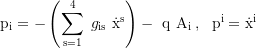Total time dilation of a neutral particle:Additional time dilation for a charged particle:Total time dilation of a charged particle:Relation between the first time derivatives and the covariant momentum components: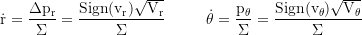Relation between the first time derivatives and the local three-velocity components: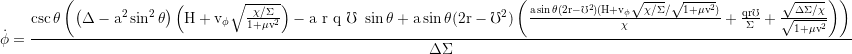with the contracted electromagnetic potential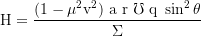The radial effective potential which defines the turning points at its zero roots isand the latitudinal potentialwith the parameterFor the 3-velocity relative to a local ZAMO we take E and solve for v:Radial escape velocity for a neutral particle:For the escape velocity of a charged particle with zero orbital angular momentum we set E=1 and solve for v:1. Constant of motion: Total energy E=-pt2. Constant of motion: axial angular momentum Lz=+pφ3. Constant of motion: Carter's constantwith the coaxial component of the angular momentum, which itself is not a constant:The azimuthal and latitudinal impact parameters areGravitative time dilatation of a corotating neutral ZAMO, infinite at the horizon:Time dilation of a stationary particle, infinite at the ergosphere:Frame-dragging angular velocity observed at infinity:Local frame-dragging velocity relative to the fixed stars (c at the ergosphere):with the relationAxial and coaxial radius of gyration:Axial and coaxial circumference: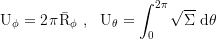The radii of the equatorial photon orbits are given implicitly by:The innermost stable orbit (ISCO) of a neutral particle is given by the polynomial:Radial coordinates of the horizons and ergospheres:Cartesian projection:Code: original / backupimages and animations by Simon Tyran, Vienna (Yukterez) - reuse permitted under the Creative Commons License CC BY-SA 4.0Simon Tyran aka Симон Тыран @ minds || vk || 1984 || wikipedia || stackexchange || wolframZurück zu „Yukterez Notepad“

Wer ist online?

Mitglieder in diesem Forum: 0 Mitglieder und 2 Gäste